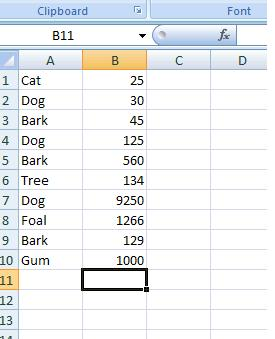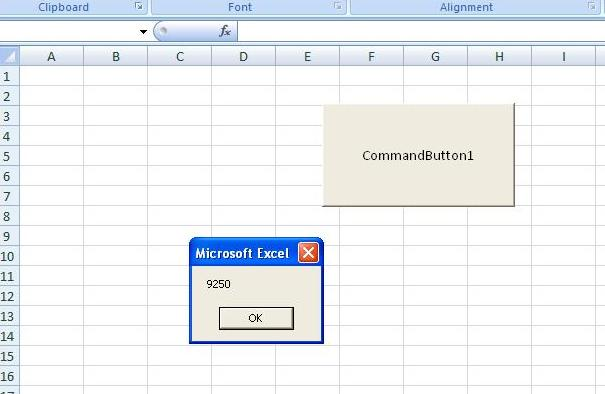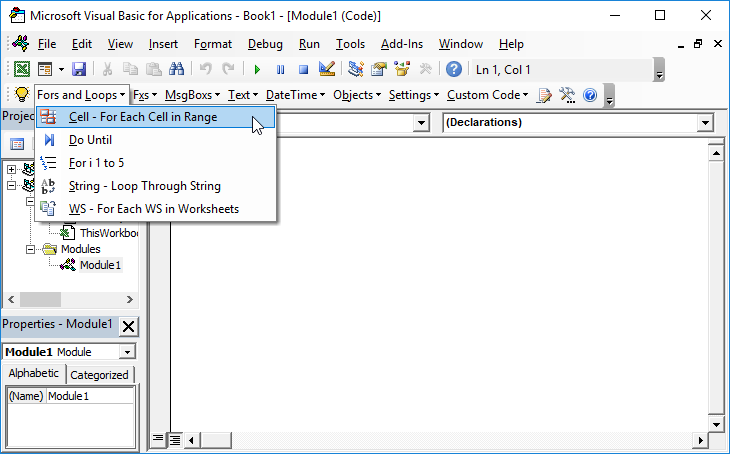# Vlookup – Multiple Results with VBA

## Vlookup Text

The standard Vlookup Function can be used to find a value within a table:And we would use VLOOKUP like so:

``=VLOOKUP("Dog",A1:B10,2,FALSE)``

to give the value 30.

However, in this list we see that Dog occurs 3 times. The standard VLOOKUP function will only return the value associated with the first item in this list. It won’t return the 125 or 9,250 with the 2nd or 3rd instance of “dog” in this list.

## Vlookup Multiple Results

The following function allows us to specify a range, an expression to be searched for, and the instance (result number) and then return the corresponding value:

``````Function Find_nth_Occurrence(Column_Range As Range, Expression As String, Occ As Integer) As Double
Dim Cell
Dim Occurrences_to_date As Integer

Find_nth_Occurrence = 1000000

Occurrences_to_date = 0

For Each Cell In Column_Range
If Cell.Value = Expression Then
Occurrences_to_date = Occurrences_to_date + 1
If Occurrences_to_date = Occ Then
Find_nth_Occurrence = Cell.Offset(0, 1).Value

End If
End If
Next Cell

End Function``````

The main difference between this and the standard VLOOKUP function is that in this case, the range is the only the range of labels – not the entire data range.
The following is a subroutine that calls this function based on the click event from a command button. It looks in the range A1:A8 on Sheet2, for the 3rd instance of the word Dog:

``````Private Sub CommandButton1_Click()
End Sub``````

The variable “Answer” stores the result of the function – which is then displayed in a Msgbox on the screen:>However if the word can’t be found in the list or the frequency does not occur e.g there isn’t a 5th instance of the word “Dog”, then the value of 1,000,000 is returned :-
Or

Stop searching for VBA code online. Learn more about AutoMacro – A VBA Code Builder that allows beginners to code procedures from scratch with minimal coding knowledge and with many time-saving features for all users!